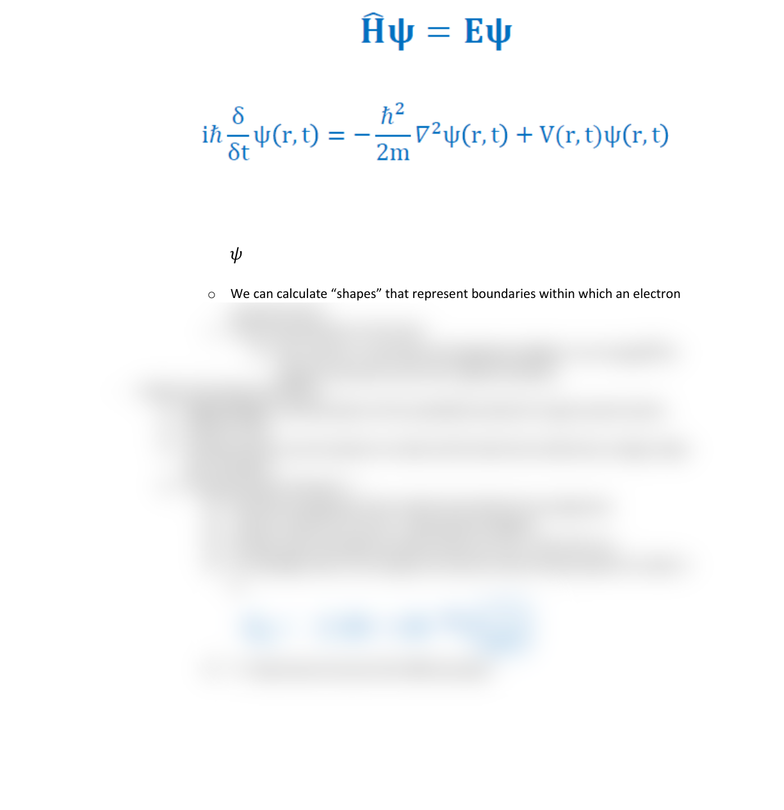Class Notes (1,100,000)
CA (650,000)
UTSC (30,000)
Chemistry (600)
CHMA10H3 (200)
Lecture 12

# CHMA10H3 Lecture Notes - Lecture 12: Azimuthal Quantum Number, Max Born, Wave Equation

Department
Chemistry
Course Code
CHMA10H3
Professor
Marco Zimmer
Lecture
12

This preview shows half of the first page. to view the full 2 pages of the document.CHMA10- Lecture 12 (Feb. 1st)
Quantum Model of the Atom
- Quantum Mechanics: The Wave Equation
Schrodinger latched onto the idea of e- as waves and in 1925 came up with differential
equation (known as Schrodinger equation) to describe an e- in an atom as a wave
Schrodinger Wave Equation (more detail)
Describes both the particle and wave nature of the e- in a hydrogen atoms
Max Born realized that he can calculate the probability density for the e- by
looking at the function 𝜓2
o 𝜓2 gives a 3D probability density plot for an electrons position in space called
orbital
o We can calculate shapes that represent boundaries within which an electron
should be found
o Visual representation of the atom:
Each electron is described by 3 quantum numbers: n for energy, for
angular momentum and m for spatial orientation
- Orbitals and Quantum Numbers
Atomic Orbital: a 3D description of the probability density for a given wave function
Orbital Orbit
Characterized by a set of quantum numbers which determine orbital size, energy, shape
and orientation
Principal Quantum Number, n
Describes average size of the orbital and indicates the energy level
n take on values of 1,2,3,4,5 (only positive integers)
Numbers also correspond to letters where 1=K, 2=L, 3=M, 4=N, etc
In a hydrogen atom, the energy of an electron with principal quantum number n
is:
n determines the size of this 90% boundary